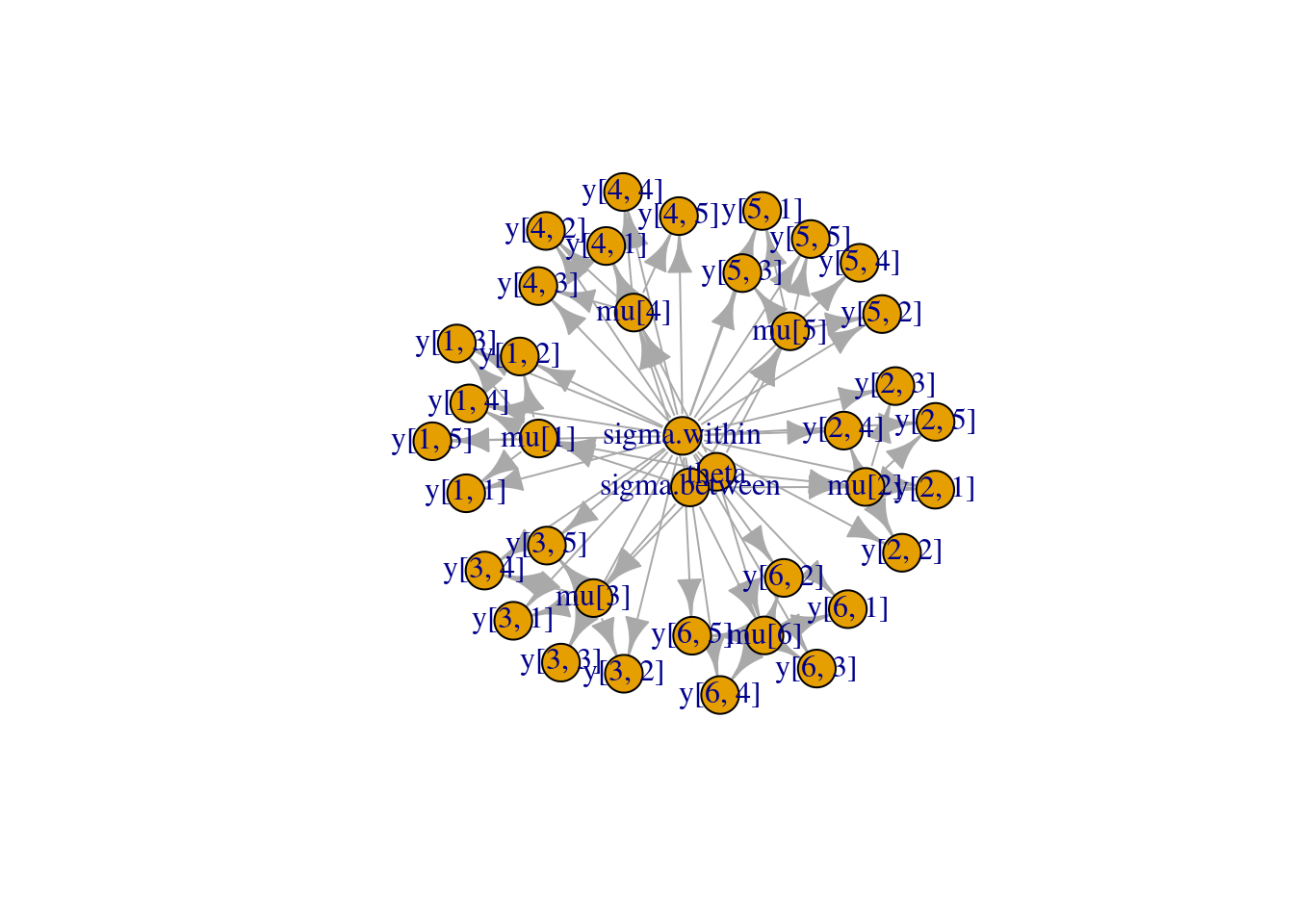# Building a model from BUGS code in R using NIMBLE

The BUGS language for declaring statistical models was popularized by WinBUGS, OpenBUGS and JAGS. Those generate and run an MCMC for the model, but they don’t allow a programmer to use the model in any other way. NIMBLE provides a new implementation of the BUGS language and creates model objects you can program with.

NIMBLE supports most models written for BUGS or JAGS. It also extends the BUGS language in a bunch of ways that we won’t go into here. See the User Manual for more details.

## Dyes example

Let’s pick a simple model from the classic WinBUGS examples: the dyes example. This is a simple normal hierarchical model. A description can be found here. A copy of the original is on our GitHub repository here. A modified version we will use is set up like this:

library(nimble, warn.conflicts = FALSE)
## nimble version 1.0.1 is loaded.
##
## Note for advanced users who have written their own MCMC samplers:
##   As of version 0.13.0, NIMBLE's protocol for handling posterior
##   predictive nodes has changed in a way that could affect user-defined
##   samplers in some situations. Please see Section 15.5.1 of the User Manual.
dyesCode <- nimbleCode({
# Model
for (i in 1:BATCHES) {
for (j in 1:SAMPLES) {
y[i,j] ~ dnorm(mu[i], sd = sigma.within);
}
mu[i] ~ dnorm(theta, sd = sigma.between);
}

# Priors
theta ~ dnorm(0.0, 1.0E-10);
sigma.within ~ dunif(0, 100)
sigma.between ~ dunif(0, 100)
})

Compared to the original, this has been modified by using standard deviation parameters instead of precision parameters in two places – to illustrate NIMBLE’s ability to handle different parameterizations – and by removing the posterior predictive nodes.

By the way, any of the standard WinBUGS examples can be loaded automatically in NIMBLE like this:

classicDyesModel <- readBUGSmodel('dyes', dir = getBUGSexampleDir('dyes'))

## Create the model

We can create a model from dyesCode like this:

dyesModel <- nimbleModel(dyesCode, constants = list(BATCHES = 6, SAMPLES = 5))
## Defining model
## Building model
## Running calculate on model
##   [Note] Any error reports that follow may simply reflect missing values in model variables.
## Checking model sizes and dimensions
##   [Note] This model is not fully initialized. This is not an error.
##          To see which variables are not initialized, use model$initializeInfo(). ## For more information on model initialization, see help(modelInitialization). And we can set data values in it like this: data <- matrix(c(1545, 1540, 1595, 1445, 1595, 1520, 1440, 1555, 1550, 1440, 1630, 1455, 1440, 1490, 1605, 1595, 1515, 1450, 1520, 1560, 1510, 1465, 1635, 1480, 1580, 1495, 1560, 1545, 1625, 1445), nrow = 6) dyesModel$setData(list(y = data))
dyesModel$y ## [,1] [,2] [,3] [,4] [,5] ## [1,] 1545 1440 1440 1520 1580 ## [2,] 1540 1555 1490 1560 1495 ## [3,] 1595 1550 1605 1510 1560 ## [4,] 1445 1440 1595 1465 1545 ## [5,] 1595 1630 1515 1635 1625 ## [6,] 1520 1455 1450 1480 1445 It is also possible to set data values when calling nimbleModel. ## Use the model Now the model is an R object, and we can manipulate it as such. ### Set or get values dyesModel$theta <- 1500
dyesModel$mu <- rnorm(6, 1500, 50) dyesModel$sigma.within <- 20
dyesModel$sigma.between <- 20 dyesModel$y[1,]
##  1545 1440 1440 1520 1580
dyesModel$theta ##  1500 ### Calculate log probability densities for part or all of the model ## arbitrary example dyesModel$calculate(c('theta', 'mu[1:6]', 'y[,2]'))
##  -251.4735

### Simulate part or all of the model

## arbitrary example
dyesModel$mu ##  1460.749 1413.188 1455.944 1460.185 1400.459 1631.845 dyesModel$simulate(c('mu[1:3]'))
dyesModel$mu ##  1533.391 1514.685 1514.199 1460.185 1400.459 1631.845 ### Query the model’s relationships ## arbitrary example dyesModel$getDependencies(c('theta', 'mu'))
##   "theta"   "mu"   "mu"   "mu"   "mu"   "mu"   "mu"
##   "y[3, 1]" "y[3, 2]" "y[3, 3]" "y[3, 4]" "y[3, 5]"

### Plot the model graph (thanks to igraph’s plot feature)

library(igraph)
##
## Attaching package: 'igraph'
## The following objects are masked from 'package:stats':
##
##     decompose, spectrum
## The following object is masked from 'package:base':
##
##     union
plot(dyesModel$getGraph())With many nodes, the automatically generated graph can be hard to interpret. Note that arrows indicate node dependencies. The mu nodes depend on the centrally plotted sigma.within, sigma.between, and theta nodes, and the ys depend on their corresponding mus. The purpose of this illustration is to visualize that NIMBLE models are represented as graphical objects that can be used programmatically. ## Compile the model Finally we can compile the model and use the compiled version the same way we used the uncompiled version above: compiled_dyesModel <- compileNimble(dyesModel) ## Compiling ## [Note] This may take a minute. ## [Note] Use 'showCompilerOutput = TRUE' to see C++ compilation details. compiled_dyesModel$theta <- 1450
compiled_dyesModel\$calculate() ## all nodes by default
##  -742.8629

Naturally, the compiled version is much faster.